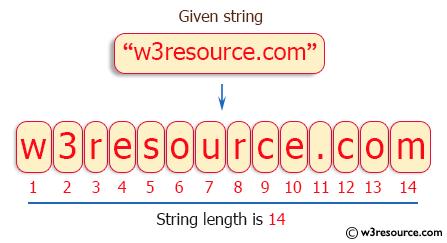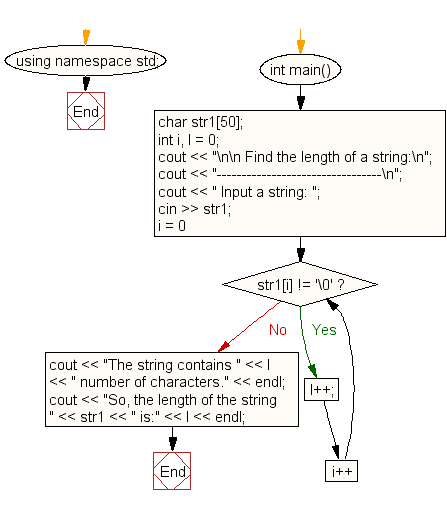﻿ C++ : Find the length of a string without library function

# C++ Exercises: Find the length of a string without using the library function

## C++ For Loop: Exercise-34 with Solution

Write a program in C++ to find the length of a string without using the library function.

Visual Presentation:Sample Solution:-

C++ Code :

``````#include <iostream> // Include the input/output stream library
#include <string>   // Include the string handling library
using namespace std; // Using standard namespace

int main() // Main function where the execution of the program starts
{
char str1; // Declare a character array to store the string
int i, l = 0; // Declare integer variables i (for iteration) and l (for counting length)

// Display message asking for input
cout << "\n\n Find the length of a string:\n";
cout << "---------------------------------\n";
cout << " Input a string: ";
cin >> str1; // Read input string from user

// Loop to count the number of characters in the string
for (i = 0; str1[i] != '\0'; i++) {
l++; // Increment the length counter for each character encountered
}

// Display the number of characters counted in the string
cout << "The string contains " << l << " number of characters." << endl;
cout << "So, the length of the string " << str1 << " is:" << l << endl;
}
``````

Sample Output:

``` Find the length of a string:
---------------------------------
Input a string: w3resource.com
The string contains 14 number of characters.
So, the length of the string w3resource.com is:14
```

Flowchart:C++ Code Editor: